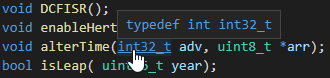# Int32_t actually 16 bit with Atmega328p and Arduino

I’m using the Atmega328p on the Arduino framework. I found out that even when explicitly using int32_t or uint32_t, the variable is actually only 16 bit. The reason is, that they are type defined to ‘unsigned int uint32_t’. The problem here, is that on the 8 bit AVR problem, ‘int’ is only 16 bit. The type defines are in ‘toolchain-atmelavr>avr>include>stdint.h’. Obviously I would expect it to be 32 bit like it is in the Arduino IDE. Is there something I am doing wrong, or is this a bug?

I’m using VS Code, PIO 5.1.1 and Atmel AVR 3.3.0

Not reproducable.

Running the code

``````#include <Arduino.h>
#define xstr(s) str(s)
#define str(s) #s

void setup(){
Serial.begin(9600);
}

#define PRINT_SIZE_OF(type) Serial.println("Size of " str(type) " is: " + String(sizeof(type)*8) + " bits");

void loop() {
PRINT_SIZE_OF(int);
PRINT_SIZE_OF(int32_t);
PRINT_SIZE_OF(uint32_t);
PRINT_SIZE_OF(int_least32_t);
PRINT_SIZE_OF(uint_least32_t);
PRINT_SIZE_OF(int64_t);
PRINT_SIZE_OF(uint64_t);
delay(1000);
}
``````

outputs

``````Size of int is: 16 bits
Size of int32_t is: 32 bits
Size of uint32_t is: 32 bits
Size of int_least32_t is: 32 bits
Size of uint_least32_t is: 32 bits
Size of int64_t is: 64 bits
Size of uint64_t is: 64 bits
``````

with the `platformio.ini`

``````[env:uno]
platform = atmelavr
board = uno
framework = arduino
``````

All fixed-width or at-least-width types work as advertised.

Using packages and versions

``````PLATFORM: Atmel AVR (3.3.0) > Arduino Uno
HARDWARE: ATMEGA328P 16MHz, 2KB RAM, 31.50KB Flash
DEBUG: Current (avr-stub) On-board (avr-stub, simavr)
PACKAGES:
- framework-arduino-avr 5.1.0
- tool-avrdude 1.60300.200527 (6.3.0)
- toolchain-atmelavr 1.70300.191015 (7.3.0)
``````

I don’t have the HW on me currently to test, but I will note, that for the board I have the ‘Atmega328p’ and not Uno and I do not have AVR dude.

I can also treat my Uno board as `board = Atmega328P` in the `platformio.ini` and the output stays the same. (from docs).

Strange, all I can currently add is this image, which indicates that int32_t is typ defined to intI tested your code on the Atmega328p and could not reproduce my error either. Turns out, I made some incorrect assumptions: the first, was that ‘typedef int int32_t’ would mean, that it just gets set to an integer aka 16 bits on the AVR platform, while I don’t quite understand why this is not the case, this was not the root of my problem. My actual problem was my second assumption: putting the whole add operation of a 16 and 32 bit integer into a single cast is the same would work. It does not… What I had to do is cast only the 16 bit integer as a 32 bit one. Rookie mistake.

Thank you max for the quick response and for the work all of you mods put in this community. I have yet to see a post without a single response as is too often the case in a lot of communities. Keep up the good work!